# # d3-interpolate

``````var i = d3.interpolateNumber(10, 20);
i(0.0); // 10
i(0.2); // 12
i(0.5); // 15
i(1.0); // 20
``````

``````d3.interpolateLab("steelblue", "brown")(0.5); // "rgb(142, 92, 109)"
``````

``````var i = d3.interpolate({colors: ["red", "blue"]}, {colors: ["white", "black"]});
i(0.0); // {colors: ["rgb(255, 0, 0)", "rgb(0, 0, 255)"]}
i(0.5); // {colors: ["rgb(255, 128, 128)", "rgb(0, 0, 128)"]}
i(1.0); // {colors: ["rgb(255, 255, 255)", "rgb(0, 0, 0)"]}
``````

## # Installing

`NPM` 安装: `npm install d3-interpolate`. 此外还可以下载 latest release (opens new window). 你可以直接从 d3js.org (opens new window) 作为 standalone library (opens new window) 或作为 D3 4.0 (opens new window) 的一部分直接引入. 支持 `AMD`, `CommonJS` 以及基本的标签引入形式。如果使用标签引入则会暴露全局 `d3` 变量:

``````<script src="https://d3js.org/d3-color.v1.min.js"></script>
<script src="https://d3js.org/d3-interpolate.v1.min.js"></script>
<script>

var interpolate = d3.interpolateRgb("steelblue", "brown");

</script>
``````

## # API Reference

# d3.interpolate(a, b)

1. 如果 b`null`, `undefined` 或者 `boolean`, 则使用常量 b.
2. 如果 b`number`, 使用 interpolateNumber.
3. 如果 bcolor (opens new window) 或可以转为颜色的字符串, 使用 interpolateRgb.
4. 如果 bdate (opens new window), 使用 interpolateDate.
5. 如果 b`string`, 使用 interpolateString.
6. 如果 barray (opens new window), 使用 interpolateArray.
7. 如果 b 可以转为 `number`, 使用 interpolateNumber.
8. 使用 interpolateObject.

# d3.interpolateNumber(a, b) <> (opens new window)

``````function interpolator(t) {
return a * (1 - t) + b * t;
}
``````

# d3.interpolateRound(a, b) <> (opens new window)

# d3.interpolateString(a, b) <> (opens new window)

# d3.interpolateDate(a, b) <> (opens new window)

# d3.interpolateArray(a, b) <> (opens new window)

# d3.interpolateObject(a, b) <> (opens new window)

# d3.interpolateTransformCss(a, b) <> (opens new window)

# d3.interpolateTransformSvg(a, b) <> (opens new window)

# d3.interpolateZoom(a, b) <> (opens new window)

## # Sampling

# d3.quantize(interpolator, n) <> (opens new window)

## # Color Spaces

# d3.interpolateRgb(a, b) <> (opens new window)Returns an RGB color space interpolator between the two colors a and b with a configurable gamma. If the gamma is not specified, it defaults to 1.0. The colors a and b need not be in RGB; they will be converted to RGB using d3.rgb (opens new window). The return value of the interpolator is an RGB string.

# d3.interpolateRgbBasis(colors) <> (opens new window)

# d3.interpolateRgbBasisClosed(colors) <> (opens new window)

# d3.interpolateHsl(a, b) <> (opens new window)# d3.interpolateHslLong(a, b) <> (opens new window)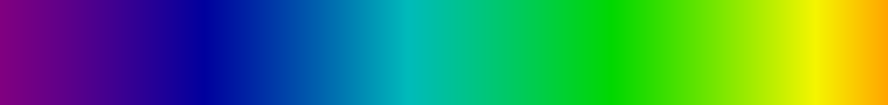interpolateHsl 类似, 但是不使用 `hues` 之间的最短路径.

# d3.interpolateLab(a, b) <> (opens new window)# d3.interpolateHcl(a, b) <> (opens new window)# d3.interpolateHclLong(a, b) <> (opens new window)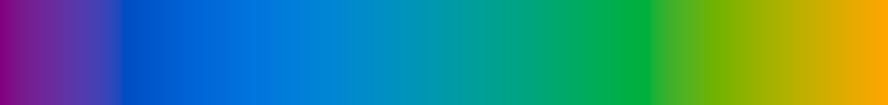interpolateHcl 类似, 但是不使用 `hues` 之间的最短路径.

# d3.interpolateCubehelix(a, b) <> (opens new window)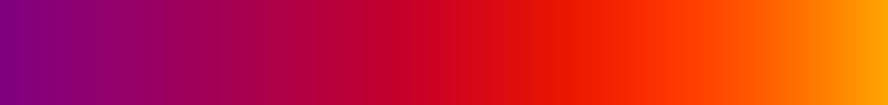# d3.interpolateCubehelixLong(a, b) <> (opens new window)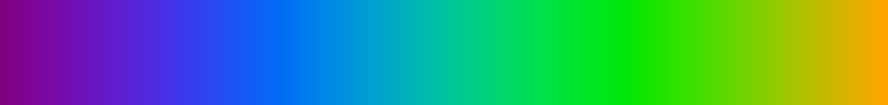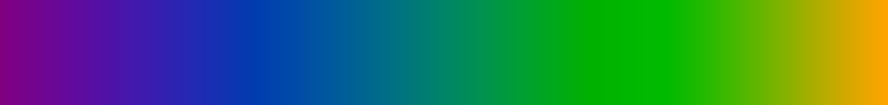interpolateCubehelix 类似, 但是不是使用两个 `hues` 值之间的最短路径.

# interpolate.gamma(gamma)

``````var interpolator = d3.interpolateRgb.gamma(2.2)("purple", "orange");
``````

## # Splines

# d3.interpolateBasis(values) <> (opens new window)

# d3.interpolateBasisClosed(values) <> (opens new window)

## # Piecewise

# d3.piecewise(interpolate, values) <> (opens new window)

``````var interpolate = d3.piecewise(d3.interpolateRgb.gamma(2.2), ["red", "green", "blue"]);
``````